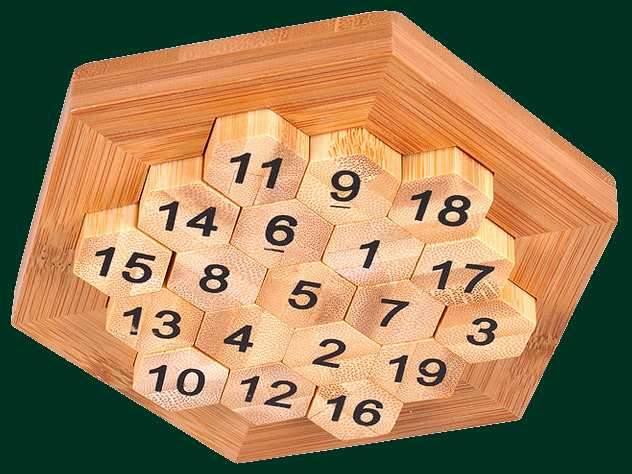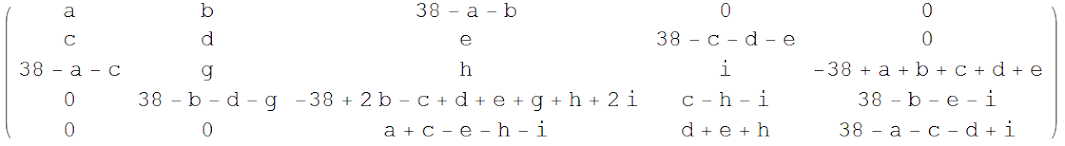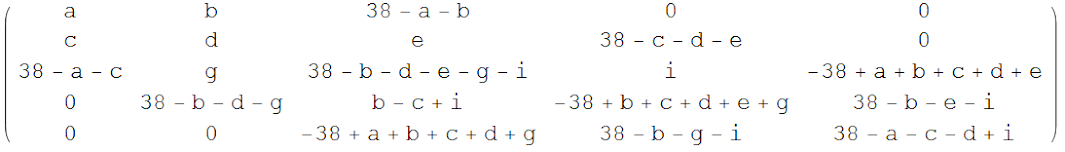## Wednesday, May 03, 2017 ...//### Aristotle's Sudoku puzzle

...and a metaphor for the exceptional structures in algebra, theoretical physics, string theory...

The most mentally demanding gift I received for our spring Xmas last night ;-) is the "Great Minds Aristotle's Number Classic Wooden Puzzle" which you may buy at amazon.com. Aristotle must have not only played with that – I think that he invented it. Unless it was invented by the Chinese because it's also sometimes named the "Lo Shu puzzle". And it may be just a modern (Chinese?) invention inspired by more primitive work of Aristotle's, I am not sure. If you hate Sudoku, you will hate this puzzle because it's Sudoku on steroids.

At those times, thinkers must have played with such wooden pieces and marbles a lot. I just verified that up to the obvious 12-element symmetry transformations, the solution to this puzzle is unique. This fact seems rather shocking. Why is the number of solutions exactly one? How could he invent it?

OK, what's the puzzle? You have a big hexagon board with 3+4+5+4+3=19 small wooden hexagon pieces in it. Those have numbers between 1 and 19 written on them. Your task is to rearrange the wooden pieces inside the big hexagon board in such a way that all the 15 sums in the rows are equal to each other.

To be sure, if you read the big hexagon as 5 rows with 3,4,5,4,3 elements, the sum of the 3 pieces in the top row must be X, the sum of the 4 pieces beneath them must be X, the sum of the 5 pieces that divide the big hexagon to halves must be X, and so on. This is five sum rules with X on the right hand side. Because the hexagon may be sliced in 3 mutually similar yet inequivalent ways (which are mutually rotated by multiples of 60 degrees), there are 15 sum rules with X on the right hand side.

OK, the first step that an intelligent third-grader as well as your humble correspondent is able to figure out is the value of X. The sum of all pieces is$1+2+\dots +19 = \frac{(1+19)\cdot 19}{2} = 190$ and because the sum of all 19 pieces is also the sum of sums of the 5 rows in a reading, we may see that$X = \frac{190}{5} = 38.$ So the sum in each of the 15 rows has to be equal to the ugly number of 38. Imagine that you assign 19 letter variables to the 19 places in the puzzle. Those 15 sum rules give you 15 conditions for these 19 variables. In fact, only 13 of these conditions are independent because "the sum of all pieces is 190" may be produced as a combination of the 15 sum rules in 3 seemingly different ways. (It should really be just 19-7=12 independent equations, not 13, I am a bit confused about it.)

OK, here is the unique solution, up to symmetries:I took this picture from the Internet. It was created by someone else so there is a mistake in the picture. See the comments for details.

You may see – but you will kindly forget – that the counterintuitive number 5 sits at the center. You could assume that there's some symmetry so the "middle number" from the set from 1 to 19, namely 10, would be in the middle. But you would be wrong. No such symmetry works here, partly because of the fact that unlike in regular Sudoku, we are constraining sums of different numbers of terms. So there is no "Y to 20-Y" counterpart of the "Y to 10-Y" reflection symmetry for the numbers.

Most of the large numbers – all the two-digit numbers – sit on the boundary of the hexagon. It's because the boundary is where the rows with only 3 elements exist, and those must still produce the sum of 38 which means that the average piece must have the value of 12.67 over there. The central 7 pieces are the numbers 1-8 with the exception of 3 which is the smallest piece that sits at the boundary – on a corner of the hexagon, if you want to know it exactly.

How did I check that it's the unique solution?

Well, I wrote the whole board in terms of 7-8 variables in Mathematica and tried all possibilities by brute force. ;-) Well, I used the symmetry group with 12 elements as well as some of the inequalities to reduce the required time to several minutes. Then I was satisfied. You really don't want to try all sequences of 7 numbers between 1 and 19 because 197 is almost a billion. Even more obviously, you don't want to try all permutations of 19 pieces because 19! exceeds 1017. So some optimization was done – I could perform a better optimization but the time became manageable.

Mathematica commands

First, I modified the shape of the hexagon to one of the 5 x 5 square matrix with 3 zeroes in the upper right corner and 3 zeroes in the opposite corner of the matrix:

mf[a_, b_, c_, d_, e_, g_, h_, i_] := {{a, b, 38 - a - b, 0, 0},
{c, d, e, 38 - c - d - e, 0},
{38 - a - c, g, h, i, -38 + a + b + c + d + e},
{0, 38 - b - d - g, -38 + b + d + g - c + h + i + b + e + i,
c - i - h, 38 - b - e - i},
{0, 0, -e - h + a + c - i, d + e + h, 38 - a - c - d + i}};
mf[a, b, c, d, e, g, h, i] // MatrixForm
I manually calculated the remaining pieces in terms of the independent a,b,c,d,e,g,h,i variables so that the sums I could see were equal to 38. OK, the source looks unreadable but in the matrix form, the output is a bit more comprehensible:Click at the matrix above to zoom it in. Then I verified whether all the 15 sums are equal to 38:
m = mf[a, b, c, d, e, g, h, i];
Total[m]
Total[Transpose[m]]
{m[[1, 3]] + m[[2, 4]] + m[[3, 5]],
m[[1, 2]] + m[[2, 3]] + m[[3, 4]] + m[[4, 5]],
m[[1, 1]] + m[[2, 2]] + m[[3, 3]] + m[[4, 4]] + m[[5, 5]],
m[[2, 1]] + m[[3, 2]] + m[[4, 3]] + m[[5, 4]],
m[[3, 1]] + m[[4, 2]] + m[[5, 3]]}
The output was
{38, 38, b + d + e + g + h + i, 38, 38}
{38, 38, b + d + e + g + h + i, 38, 38}
{38, 38, 38, -38 + 2 b + 2 d + 2 e + 2 g + 2 h + 2 i,
76 - b - d - e - g - h - i}
You see that many of them are equal to 38 automatically but some of them depend on the variables. All those will be equal to 38 assuming$b + d + e + g + h + i = 38$ Fine. So I decided to substitute $h=38-b-d-e-g-i$.
nf[a_, b_, c_, d_, e_, g_, i_] :=
mf[a, b, c, d, e, g, 38 - b - d - e - g - i, i];
n = nf[a, b, c, d, e, g, i];
n // MatrixForm
Total[n]
Total[Transpose[n]]
The matrix "n" only depends on seven variables:Also, it's interesting that if you try to compute the sums of powers of all these elements by the commands
Total[Total[n]]
Total[Total[n]*Total[n]]
Total[Total[n]*Total[n]*Total[n]]
you will get the answers 190, 7220, 274360 which are independent of the variables. If you thought that the constraints on the "standard deviations of the pieces" etc. will give you new, nonlinear equations, you would be wrong. The 15 sum rules actually guarantee that all these sums of powers are equal to the sums of powers of the numbers 1...19.

Before we calculate, it's useful to write the command
Dynamic[{a, b, c}]
which will dynamically show us how far the hard calculation has gotten. Finally, we may run the code that takes several minutes and tries all the possibilities:
For[a = 1, a <= 14, a++,
bmax = Min[37 - a - a, 19];
For[b = 19 - a, b <= bmax, b++,
cmin = Max[b + 1, 19 - a];
cmax = Min[37 - a - a, 19];
For[c = cmin, c <= cmax, c++,
For[d = 1, d <= 19, d++,
emin = Max[1, 39 - b - c - d];
emax = Min[19, 37 - c - d];
For[e = emin, e <= emax, e++,
gmin = Max[1, 39 - b - c - d];
gmax = 19 - a - b - c - d;
For[g = gmin, g <= 19, g++,
imin = Min[a + a + c + d - 39, 1, 19 - b - d - g];
imax = Max[19, 37 - b - d - g];
For[i = imin, i <= imax, i++,
If[Length[Union[{a, b, c, d, e, g, i}]] < 7, 0,
nsubstitute = nf[a, b, c, d, e, g, i];
uni = Union[Flatten[nsubstitute]];
If[uni == {0, 1, 2, 3, 4, 5, 6, 7, 8, 9, 10, 11, 12, 13, 14, 15, 16, 17, 18, 19},
Print[MatrixForm[nsubstitute]], 0];
];
]]]]]]];
Here I simply tried all values for a,b,c,d,e,g,i between 1 and 19 except that the intervals were truncated in order to speed up the calculation:
• I imposed the condition that the left upper corner variable "a" is the smallest one among the six similar corners. In this way, I gauge fixed the symmetry $\ZZ_6$ rotating the hexagon by multiples of 60 degrees – which obviously produces equivalent solutions. In this way, the time was reduced to one-sixth.
• I imposed the condition that the variable "b" is smaller than "c". In this way, I gauge fixed the $\ZZ_2$ symmetry flipping the hexagon with respect to the axis around "a". In combination with the previous point, I gauge fixed the whole symmetry of the hexagon – which is a semidirect product of a $\ZZ_6$ and a $\ZZ_2$ and has 12 elements (and it is known as the dihedral group $D_6$). The time was reduced by a factor of 12 in total.
• I reduced several intervals for the 7 variables from 1...19 to a narrower interval by imposing the condition that some of the entries in the matrix that are expressed as complicated sums or differences of variables (and/or plus minus 38) are between 1 and 19, too.
• Before I verified whether all numbers 1...19 are represented in the matrix, I checked whether the numbers a,b,c,d,e,g,i are all different from each other, and if they are not, I avoided the verification of the full matrix. I don't know whether this two-step decomposition of the task sped up the calculation substantially.
After the code was running for several minutes, it produced the unique solution:$\left( \begin{array}{ccccc} 3 & 17 & 18 & \circ & \circ \\ 19 & 7 & 1 & 11 & \circ \\ 16 & 2 & 5 & 6 & 9 \\ \circ & 12 & 4 & 8 & 14 \\ \circ & \circ & 10 & 13 & 15 \\ \end{array} \right)$ Not bad. Note that 3 is the smallest number among the 6 corner numbers 3,18,9,15,10,16 while its horizontal neighbor 17 is smaller than the vertical neighbor 19, as we required. For the sake of readability, I replaced 0 in the corners with $\circ$ – I did so in our linear algebra textbook more than two decades ago, too.

I am not sure whether I would be able to solve – let alone invent – the puzzle without the help of a computer. To remember some features of the diagram – like 3 in the corner, 5 in the center, 15 on the opposite side of 3, "3,17,18" on one edge, or something like that – seems to be the only feasible way for me to be able to solve the puzzle in the future, without the help of a computer.

Now, a deeper point. This is of course recreational mathematics but even though the solution is unique, the solution looks remarkably "symmetry-breaking" and "non-obvious". The 12-15 sum rules underdetermine the 19 variables but the condition that each number between 1 and 19 is represented exactly once happens to exactly compensate for this shortage of conditions.

In some sense, the regular Sudoku looks like the families of $U(N)$ groups. This Aristotle's Sudoku is more similar to an exceptional group. It doesn't seem to follow any "easy template" or "trivial logic" but it still happens to exist and, in fact, be the unique solution to some rather natural conditions. Exceptional, unexpected, unique solutions to conditions play a rather important role in mathematics, group theory, theoretical physics, grand unification, and string theory, among related corners of physics and mathematics.

With a lot of brute force, one may solve this Aristotle's Sudoku puzzle – and also find finite sporadic groups such as the monster group or the exceptional $E_8$ Lie group. But because of the uniqueness or near uniqueness of these structures, one is tempted to think that there must always exist a really simple, nearly spiritual explanation of the existence of these structures and solutions. The $E_8$ is the gauge group on the boundary of M-theory's spacetime (and therefore in a heterotic string theory, too). We know that it works, why it works, numerous anomaly cancellation conditions and other good news that the group obeys. But isn't there some "really simple" explanation why such a group exists and sits on the M-theory's boundaries?

Similarly, why does the monster group appear as the discrete group in the CFT dual to the pure AdS3 gravity? And if I make a jump from these physically justifiable questions, I may ask a more speculative one: Is there a solution to (or compactification of) string theory that depends on the existence of this solution to Aristotle's Sudoku puzzle?

Bonus: Finally, I realized why there are just 12 independent "sum rule" equations. There are 15 to start with. But there are 3 ways of combining the rows to get "the sum of 19 pieces is 190" which reduces 15 to 13. But there is one more redundance. There are 6 centers of the edges, 19,17,11,14,13,12. It is no coincidence that if you divide them to 2 triangles – 19,11,13 and 17,14,12 – the sums of both groups are equal (it's not easy to see why it has to be 43). The combination 19+11+13-17-14-12 may actually be obtained in two ways. You either sum the edge rows with alternating signs; or you sum the "second rows" with alternating signs (one big internal triangle minus the opposite one).

My current way of remembering the right setup is: a 3 in a corner and 17,19 as neighbors. 5 at the center. 15 in the corner against 3 – like if the solution wants to say that 3*5 = 15. 43 as the two sums of the triangles at center of the edges. 9 in another corner, the only other 1-digit piece in a corner. There are two possibilities where to place it. With these pieces, the completion of the task is almost straightforward – at most, one tries two possibilities about twice.